# 3 Laplacian eigenmaps and spectral clustering¶

In this notebook we are going to explore a graph of political similarity among members of the 50th legislature of the Swiss National Council.

In :
import utils
import numpy as np
import pandas as pd
import pygsp
import matplotlib as mpl
import matplotlib.pylab as plt

from sklearn.cluster import KMeans


The code below loads data from the ../data/ folder and formats it in the following manner:

• The dataframe council_df contains the names of each council member, their party affiliation and a color, attributed according to said party affiliation.
• The numpy array adjacency constains the adjacency matrix of the graph connecting members that have had similar voting patterns. The more similarly two members voted in previous sessions (either for or against a certain bill), the larger the weight of their connection. This adjacency matrix thusly encodes, to some degree, the political similarities in the Council.

Run the code below to inspect the head of the council_df dataframe.

In :
council_df, adjacency = utils.preprocess_swiss_council()

Out:
CouncillorName node_idx CouncillorId PartyAbbreviation Color
0 Thorens Goumaz Adèle 0 3907 PES forestgreen
1 Marra Ada 1 3923 PSS r
2 Glauser-Zufferey Alice 2 3883 UDC royalblue
3 Moser Tiana Angelina 3 3897 pvl g
4 Rickli Natalie 4 3902 UDC royalblue

## 3.1 The Laplacian matrix¶

As a first step towards investigating Laplacian eigenmaps and Spectral Clustering, take the adjacency matrix $A$ represented as the variable adjacency above and compute its corresponding combinatorial and normalized Laplacian matrices.

Now compute the eigendecomposition of these Laplacian matrices. Hint: you can use the eigensolvers in numpy.linalg.

In :
G = pygsp.graphs.Graph(W=adjacency)
G.compute_laplacian(lap_type='normalized')
# G.compute_laplacian(lap_type='combinatorial') # For the combinatorial
eigvals, eigvecs = np.linalg.eigh(G.L.toarray())


## 3.2 Laplacian eigenmaps¶

Based on the eigendecomposition you have just performed above, assign $x$ and $y$ coordinates to the Laplacian eigenmap embedding corresponding to each of the two Laplacian matrices you had before.

In :
x_coords = eigvecs[:,1]
y_coords = eigvecs[:,2]


Now, before we check how suitable these Laplacian eigenmaps embeddings is for this dataset, we display bellow a scatter plot of the Swiss National Council based on random $x$ and $y$ coordinates. Each dot represents a member of the council, color coded according to their party affiliations.

In :
# Random embedding of the Swiss National Council
utils.plot_council_with_party_colors(council_df,
x_coords=np.random.randn(len(council_df)),
y_coords=np.random.randn(len(council_df)))
plt.show()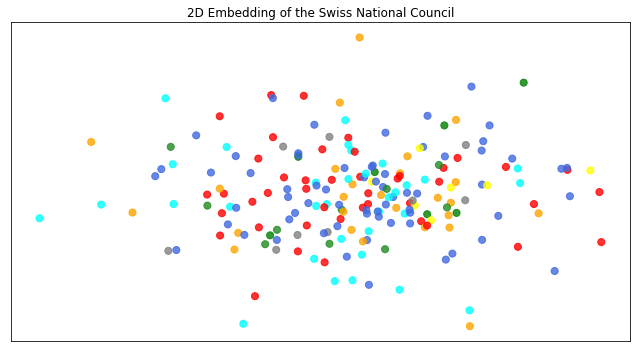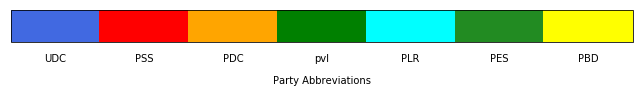Preferably using the same plotting function as above, plot your embeddings by replacing the $x$ and $y$ coordinates by those obtained via your Laplacian eigenmaps. Are your embeddings more coherent with the party structure? Which of the two Laplacian matrices produces the best embedding in your opinion?

Note that UDC is a right-wing party, PSS is a left-wing party, and PDC, pvl and PLR are considered to be more centrist. Are those observations visible in the produced embeddings?

In :
utils.plot_council_with_party_colors(council_df,
x_coords=x_coords,
y_coords=y_coords)
plt.show()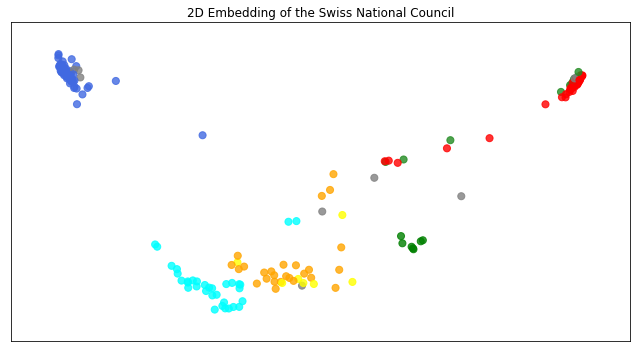## 3.3 Spectral clustering¶

In this section we will pretent not to have the party affiliation of each member and try to retrieve them (or some correlated signal) via spectral clustering of the members' graph.

As a refresher, spectral clustering consists in using the "lower" eigenvectors of the Laplacian matrix as feature vectors, which are then passed to a k-means algortithm that returns cluster assignments to each node. For what follows, you can restrict yourself to using only the normalized Laplacian eigenvectors.

For starters, perform spectral clustering on the dataset setting $k = 2$ clusters and $d=2$ eigenvectors. Hint: you can use the KMeans function from scikit-learn.

In :
cluster_assignments = KMeans(n_clusters=2,random_state=0).fit(eigvecs[:,0:3])


Now plot the cluster assignments as a color signal on the Laplacian eigenmaps embedding, in a similar way as we have done before with the party color codes. You can use the same utils.plot_council_with_party_colors functions; it has an argument custom_colors to which you can pass the cluster assignment labels (the latter is accessible via cluster_assignments.labels_ if you used sklearn.cluster.KMeans).

In :
utils.plot_council_with_party_colors(council_df,
x_coords=x_coords,
y_coords=y_coords,
custom_colors=cluster_assignments.labels_)
plt.show()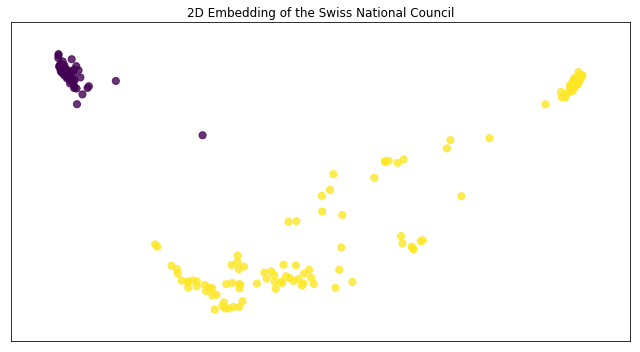Repeat this process a couple more times choosing other numbers of $k$ clusters and $d$ features and see if you can find some meaning in the retrieved cluster assignments by relating them to the party colors in the embeddings seen in Section 3.2.

Finally, set $k = 7$ and try to find the number of features $d$ that gets the spectral clustering assigment closest to the political party partition of Section 3.2.

In :
cluster_assignments = KMeans(n_clusters=7,random_state=0).fit(eigvecs[:,0:7])

utils.plot_council_with_party_colors(council_df,
x_coords=x_coords,
y_coords=y_coords,
custom_colors=cluster_assignments.labels_)
plt.show()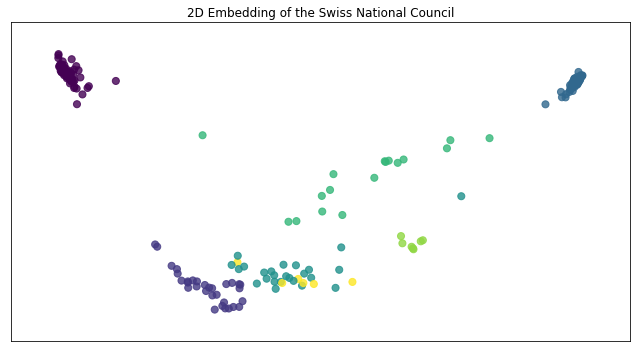In [ ]: